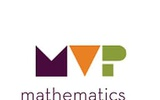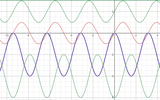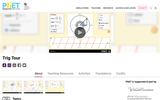Updating search results...

# 17 Results

View
Selected filters:
• NC.M3.F-TF.2Conditional Remix & Share Permitted
CC BY-NC-SA
Rating
0.0 stars
Overview:

Students explore the historical context of trigonometry as motion of celestial bodies in a presumed circular arc.
Students describe the position of an object along a line of sight in the context of circular motion.
Students understand the naming of the quadrants and why counterclockwise motion is deemed the positive direction of turning in mathematics.

Subject:
Math 3
Material Type:
Lesson
Author:
EngageNY
02/23/2020Conditional Remix & Share Permitted
CC BY-NC-SA
Rating
0.0 stars
Overview:

Students will define sine and cosine as functions for all real numbers measured in degrees.
Students will evaluate the sine and cosine functions at multiples of 30 and 45.

Subject:
Math 2
Material Type:
Lesson
Author:
EngageNY
02/23/2020Conditional Remix & Share Permitted
CC BY-NC-SA
Rating
0.0 stars
Overview:

Students define the tangent function and understand the historic reason for its name.
Students use special triangles to determine geometrically the values of the tangent function for 30°, 45°, and 60°.

Subject:
Math 3
Material Type:
Lesson
Author:
EngageNY
02/23/2020Conditional Remix & Share Permitted
CC BY-NC-SA
Rating
0.0 stars
Overview:

Students define the secant function and the co-functions in terms of points on the unit circle. They relate these names for these functions to the geometric relationships among lines, angles, and right triangles in a unit circle diagram.
Students use reciprocal relationships to relate the trigonometric functions and use these relationships to evaluate trigonometric functions for multiples of 30, 45, and 60 degrees.

Subject:
Math 3
Material Type:
Lesson
Author:
EngageNY
02/23/2020Conditional Remix & Share Permitted
CC BY-NC-SA
Rating
0.0 stars
Overview:

Subject:
Math 3
Material Type:
Lesson
Author:
The Mathematics Vision Project
03/13/2020Conditional Remix & Share Permitted
CC BY-SA
Rating
4.0 stars
Overview:

Based on knowledge of the unit Circles, students see how sinusoids (Sine and coSine waves) get their shapes.

Subject:
Geometry
Material Type:
Simulation
Provider:
GeoGebra
Provider Set:
GeoGebraTube
04/26/2012Unrestricted Use
CC BY
Rating
0.0 stars
Overview:

This is an exploration activity for students to visually see the graph of trig functions and understand about sin and cos functions and understand the concept of amplitude.

Subject:
Mathematics
Math 3
Pre-Calculus
Trigonometry
Material Type:
Activity/Lab
Author:
VIJAYALAKSHMI SANKARAN
05/27/2020Rating
3.0 stars
Overview:

Students visualize radian measures of angles traversed counter clockwise around the unit circle in this interactive resource.

Subject:
Math 3
Material Type:
Interactive
Provider:
MathsIsFun.com
Author:
Math Is Fun
02/26/2019Rating
3.0 stars
Overview:

Students will learn to use mathematical models to represent real life situations. In particular, they will use tables and equations to represent the relationship between the number of revolutions made by a "driver" and a "follower" (two connected gears in a system), and they will explain the significance of the radii of the gears in regard to this relationship.

Subject:
Math 1
Math 3
Material Type:
Lesson Plan
Provider:
Annenberg Learner
Author:
Annenberg Learner
02/26/2019Conditional Remix & Share Permitted
CC BY-NC-SA
Rating
0.0 stars
Overview:

In the previous task we had to write multiple expressions to represent Carlos’ height on a Ferris wheel, depending upon which quadrant of the wheel he was located in. Our definition of sine needs to be modified if we want to use a single expression to represent Carlos’ height at every point on the wheel. The purpose of this task is to introduce a new definition of the sine function, based on an angle of rotation approach. This is the first extension of the definition of trigonometry—to define sine (and later, cosine and tangent) so the definitions apply to angles of rotation, and not just to the acute angles of right triangles.

Subject:
Math 3
Material Type:
Lesson
Author:
The Mathematics Vision Project
03/13/2020Conditional Remix & Share Permitted
CC BY-NC-SA
Rating
0.0 stars
Overview:

The purpose of this task is to provide an opportunity for students to practice the ideas, strategies and representations that have surfaced and been examined in the previous tasks, 6.1-6.4. In the context of describing the periodic motion of the shadow of a rider on the Ferris wheel as the shadow moves back and forth across the ground when the sun is directly overhead, students will recognize that the cosine function can be used to measure the distance horizontally from the center of the wheel and they will derive the function horizontal position of the shadow = 25cos(18t).

Subject:
Math 3
Material Type:
Lesson
Author:
The Mathematics Vision Project
03/13/2020Rating
0.0 stars
Overview:

Students learn about parallax in this Moveable Museum unit, in which they use mathematical techniques related to parallax to calculate the height of an object. The eight-page PDF guide includes suggested general background readings for educators, activity notes, step-by-step directions, a Data Sheet and a Tangent Table, and an astrolabe template.

Subject:
Science
Material Type:
Activity/Lab
Provider:
American Museum of Natural History
07/31/2019Unrestricted Use
CC BY
Rating
3.0 stars
Overview:

Description of cosine function with image, calculation of length of hypotenuse with known angle and adjacent side, calculation of angle with known adjacent and hypotenuse sides. Explanation of inverse cosine. Interactive fill in the blank of main points.

Subject:
Geometry
Material Type:
Interactive
Provider:
Michigan Virtual
07/19/2019Conditional Remix & Share Permitted
CC BY-NC-SA
Rating
0.0 stars
Overview:

While previous tasks have solidified students’ understanding of radians as the ratio of the length of an intercepted arc to the radius of the circle on which that arc lies, this task builds a new understanding of radians —the radian measure of an angle is the length of the intercepted arc on a unit circle. Students will also use radians, rather than degrees, to find trigonometric values for angles measured in radians, and will observe that the x and y-coordinates of points on the unit circle correspond with the sine and cosine of the angle of rotation measured from the ray passing through the point (1, 0).

Subject:
Math 3
Material Type:
Lesson
Author:
The Mathematics Vision Project
03/13/2020Conditional Remix & Share Permitted
CC BY-NC-SA
Rating
0.0 stars
Overview:

The purpose of this task is to solidify students’ previous understanding of radians—that is, the radian measure of an angle is the ratio of the length of an intercepted arc to the radius of the circle on which that arc lies, and to use radian measurement as a proportionality constant in computations. This task and the previous task lay a foundation for a new understanding of radians —that is, the radian measure of an angle is the length of the intercepted arc on a unit circle. This latter idea will be solidified in the next task of this learning cycle.

Subject:
Math 3
Material Type:
Lesson
Author:
The Mathematics Vision Project
03/13/2020Unrestricted Use
CC BY
Rating
0.0 stars
Overview:

Sample Learning Goals
Define trig functions for negative angles and angles greater than 90 degrees.
Translate between multiple representations of trig functions: as sides of a right triangle inscribed in a unit circle, graph of the function vs. angle, and numerical values of the function.
Deduce the sign (+, -, 0) of a trig function for any given angle without a calculator using the unit circle concept.
Estimate the value of trig functions for any given angle without a calculator using the unit circle concept.
Define exact trig functions for special angles using degrees or radians for angle measures.

Subject:
Science
Material Type:
Activity/Lab
Author:
PhET Interactive Simulations
10/05/2021Conditional Remix & Share Permitted
CC BY-NC-SA
Rating
0.0 stars
Overview:

The purpose of this task is to practice finding the sine and cosine of angles of rotation on the unit circle when the angle is measured in radians counterclockwise from the ray passing through the point (1, 0); that is, angles drawn in standard position.

Subject:
Math 3
Material Type:
Lesson
Author:
The Mathematics Vision Project